### Junior Balkan MO 2012

Kvaliteta:
Avg: 2,0
Težina:
Avg: 3,0
Let the circles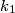$k_1$ and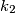$k_2$ intersect at two points$A$ and$B$, and let t be a common tangent of$k_1$ and$k_2$ that touches$k_1$ and$k_2$ at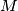$M$ and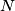$N$ respectively. If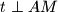$t\perp AM$ and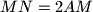$MN=2AM$, evaluate the angle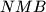$NMB$.